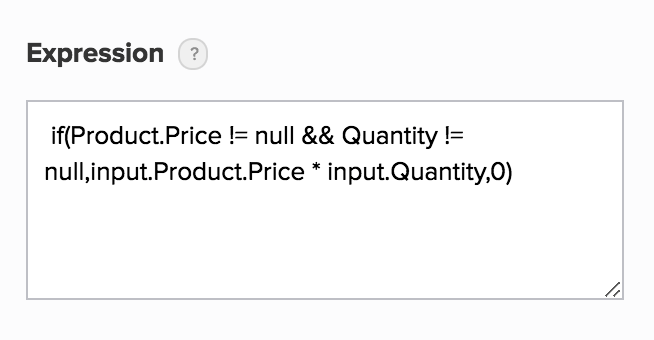# Set expression for formula field

1. Open the form builder.
2. Select the required formula field.
3. Navigate to the Field Properties pane on the right.
4. Click the input box below Expression. The Calculate Expression pop-up window will appear.
5. Set the required Expression:
• You can include fields in the expression by either selecting them (from the Refer Fields column) or typing their field link names in the Expression box. You can include all field types except add notes and section.
• You can include Deluge System Variables by typing them
• You can use built-in functions by typing them
6. Click Insert conditional formula to define one. Learn how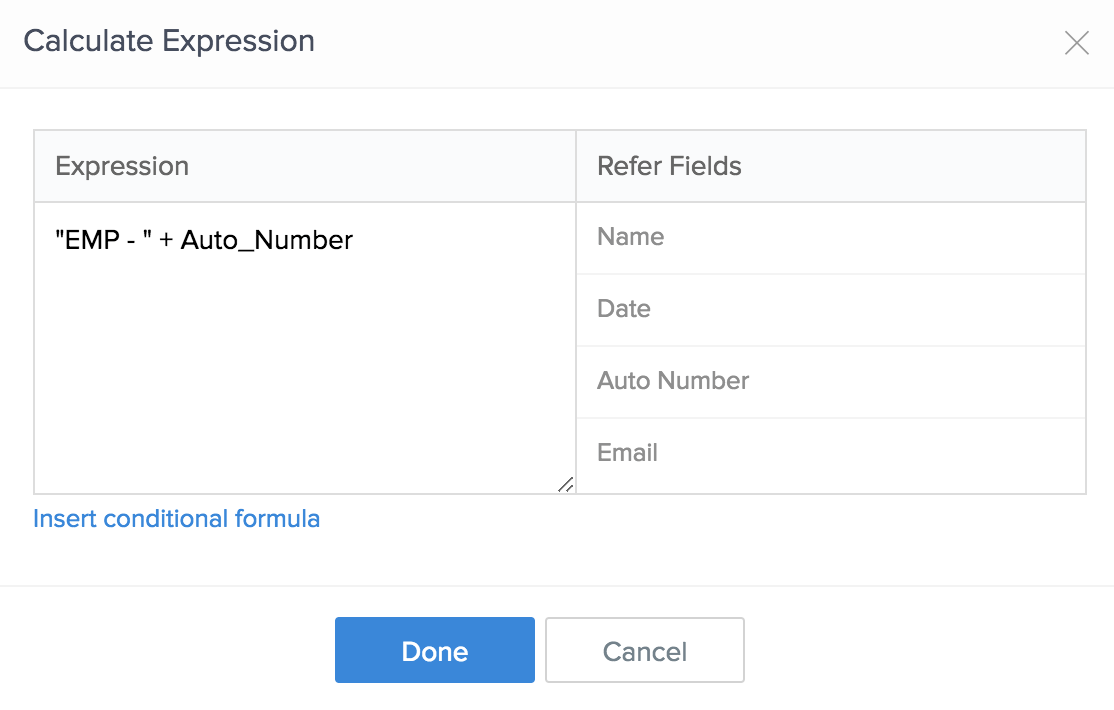7. Upon setting the required Expression, click Done. The expression you set will then appear in the input box below Field Properties > Expression.​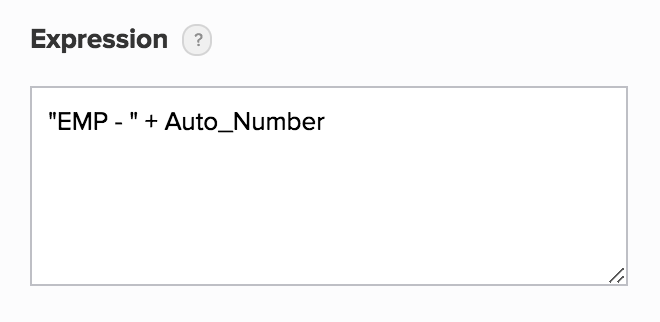## Set a conditional expression

1. Open the form builder.
2. Select the required formula field.
3. Navigate to the Field Properties on the right.
4. Click the input box below Expression. The Calculate Expression pop-up window will appear.
5. Click Insert conditional formula.
6. Set the Condition
• It must be a boolean expression — an expression which evaluates to true if it is logically correct, else false
• You can refer to the fields by typing their field link names
• You can use logical functions by typing them
7. Set Value1 and Value2:
• ​These can either be static values or expressions
• The formula field is assigned with Value1 when the specified Condition evaluates to true
• The formula field is assigned with Value2 when the specified Condition evaluates to false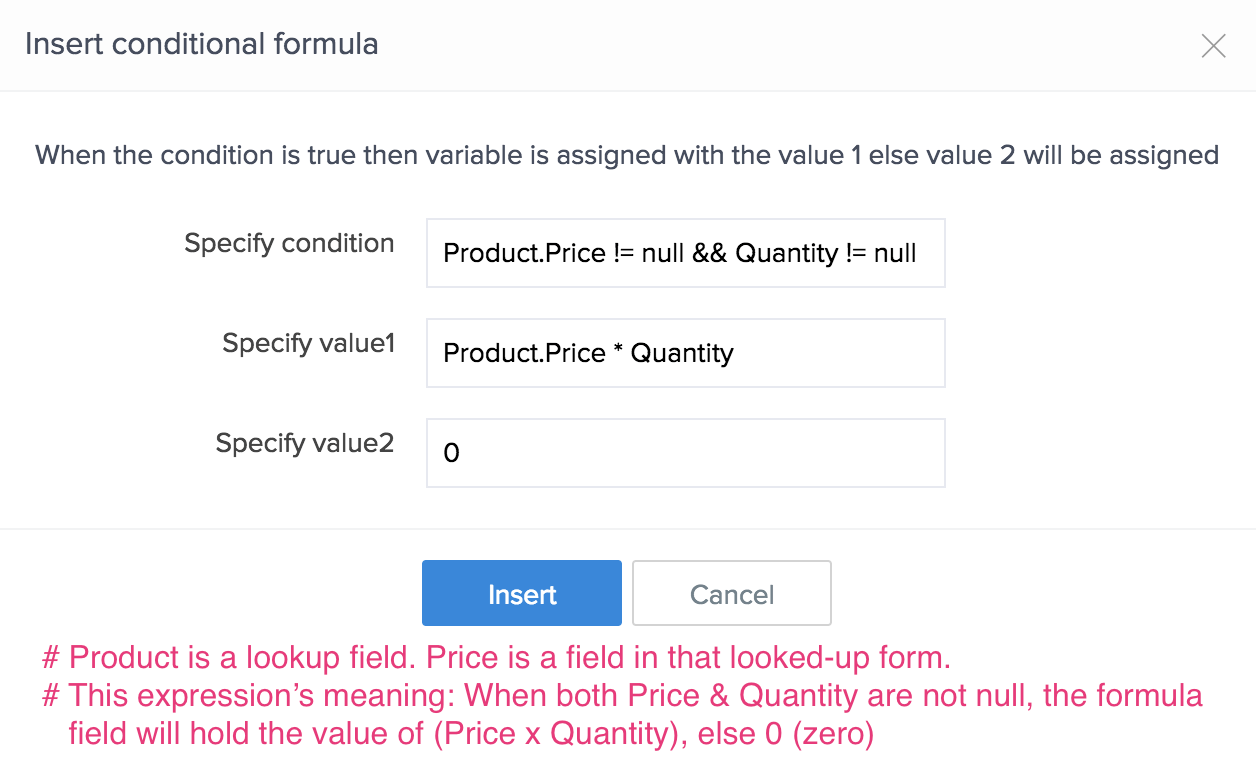8. Click Insert. The conditional expression will apper in the Calculate Expression pop-up window.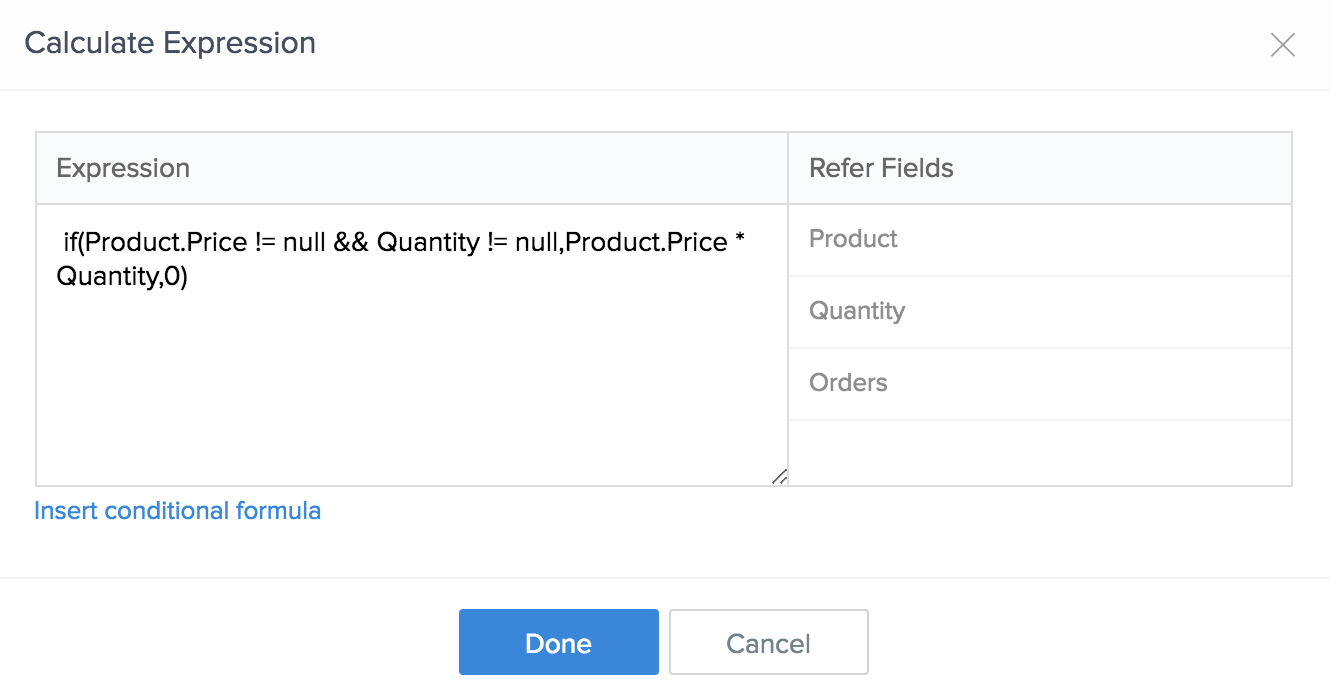9. Click Done. The expression will then appear in the input box below Field Properties > Expression.​